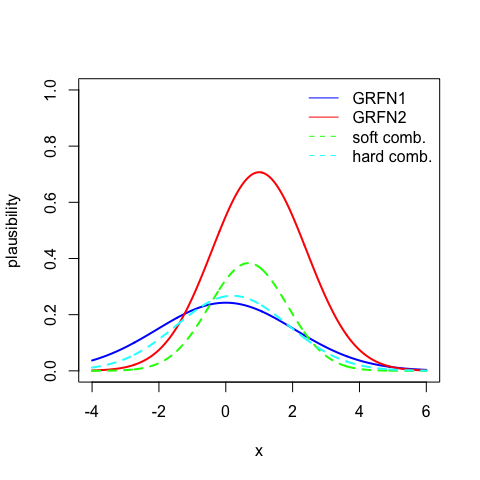# Introduction to the evreg package

#### 2023-04-04

The evreg package implements ENNreg (Denœux 2022) (Denœux 2023b), a neural network model for regression in which prediction uncertainty is quantified by Gaussian random fuzzy numbers (GRFNs), a newly introduced family of random fuzzy subsets of the real line that generalizes both Gaussian random variables and Gaussian possibility distributions (Denœux 2023a). The output GRFN is constructed by combining GRFNs induced by prototypes using a combination operator that generalizes Dempster’s rule of Evidence Theory. The three output units indicate the most plausible value of the response variable, variability around this value, and epistemic uncertainty. The network is trained by minimizing a loss function that generalizes the negative log-likelihood.

The evreg package contains functions for training the ENNreg model in batch or online mode, tuning hyperparameters by cross-validation or the hold-out method, and making predictions. It also contains utilities for making calculations with GRFNs (such as, e.g., computing the degrees of belief and plausibility of an interval, or combining GRFNs).

The user is invited to read the papers cited in this vignette to get familiar with the main concepts underlying epistemic random fuzzy sets and evidential regression. These papers can be downloaded from the author’s web site, at https://www.hds.utc.fr/~tdenoeux/. Here, we provide a short guided tour of the main functions in the evreg package.

You first need to install this package:

library(evreg)

The following sections contain a brief introduction on the way to use the main functions in the package evreg for evidential regression.

## Evidential regression

### Data generation

Let us start by writing a function that generates a dataset similar to that used in Section V.A of (Denœux 2023b):

gendat<-function(n){
x<-rep(0,n)
y<-x
for(i in 1:n){
u<-runif(1)
if(u<0.5) x[i]<-runif(1,-3,-1) else x[i]<-runif(1,1,4)
if(x[i]<0) y[i] <- sin(3*x[i])+ x[i]+ sqrt(0.01)* rnorm(1)
else y[i] <- sin(3*x[i])+ x[i]+ sqrt(0.3)* rnorm(1)
}
return(list(x=x,y=y))
}

We generate training and test sets of sizes, respectively, 400 and 1000:

n<-400
nt<-1000
set.seed(20230104)
train<-gendat(n)
test<-gendat(nt)

### Hyperparameter tuning and learning

Let us determine hyperparameters $$\xi$$ and $$\rho$$ using cross-validation, with batch training and $$K=30$$ prototypes:

cv<-ENNreg_cv(train$x,train$y,K=30,XI=c(0,0.01,0.1),RHO=c(0,0.01,0.1),verbose=FALSE)
cv
## $xi ##  0.01 ## ##$rho
##  0.1
##
## $RMS ## [,1] [,2] [,3] ## [1,] 0.4903299 0.4602456 0.4544297 ## [2,] 0.4895777 0.4553594 0.4541733 ## [3,] 0.4810569 0.4592168 0.4697710 We can then train again the model using all the training data and the selected hyperparameters: fit<-ENNreg(train$x,train$y,K=30,xi=cv$xi,rho=cv$rho,verbose=FALSE) Let us now compute the predictions for regularly spaced inputs: xt<-seq(-4,5,0.01) pred<-predict(fit,xt) and let us compute belief intervals at levels 50%, 90% and 99%: int50<-intervals(pred,0.50) int90<-intervals(pred,0.9) int99<-intervals(pred,0.99) We can now plot the results using function ggplot of package ggplot2: library(ggplot2) int<-data.frame(lwr50=int50$INTBel[,1],upr50=int50$INTBel[,2], lwr90=int90$INTBel[,1],upr90=int90$INTBel[,2], lwr99=int99$INTBel[,1],upr99=int99$INTBel[,2], x=xt,mux=pred$mux)
ggplot(data=as.data.frame(train), aes(x = x)) +
geom_point(aes(y = y)) +
geom_ribbon(data=int, aes(ymin = lwr50, ymax = upr50,x=x),alpha=0.2)+
geom_ribbon(data=int, aes(ymin = lwr90, ymax = upr90,x=x),alpha=0.15)+
geom_ribbon(data=int, aes(ymin = lwr99, ymax = upr99,x=x),alpha=0.1) +
geom_line(data=int,aes(x = x,y=mux),color="red",linewidth=1.5)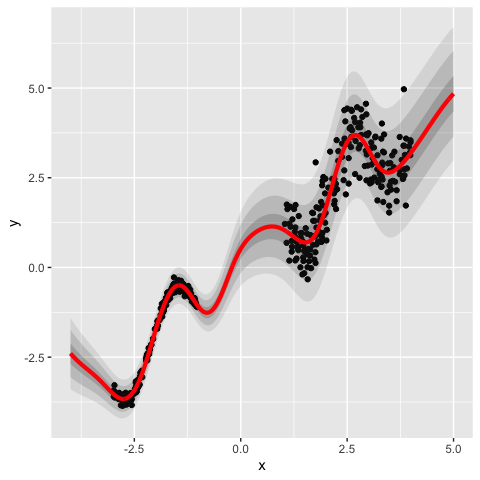### Calibration curves

Let us now plot calibration curves for probabilistic and belief prediction intervals. We start by computing the predictions for the test set:

pred.tst<-predict(fit,test$x,test$y)

We then compute prediction intervals and their coverage rates for 9 equally spaced levels between 0.1 and 0.9:

A<-seq(0.1,0.9,0.1)
nA<-length(A)
probbel<-rep(0,nA)
probp<-rep(0,nA)
for(i in 1:nA){
int<-intervals(pred.tst,A[i],test$y) probbel[i]<-int$coverage.Bel
probp[i]<-int$coverage.P } Finally, we can plot the calibration curves: oldpar <- par(pty="s") plot(c(0,A,1),c(0,probp,1),type="l",lwd=2,col="blue",xlab="",ylab="") lines(c(0,A,1),c(0,probbel,1),col="red",lwd=2) abline(0,1,lty=2) title(ylab="coverage rate", line=2.2, cex.lab=1.2) title(xlab="level", line=2.2, cex.lab=1.2)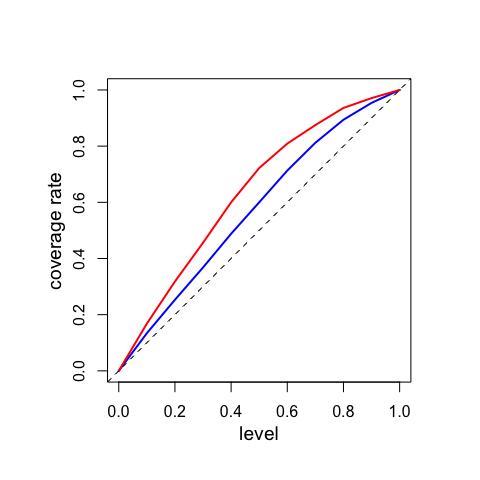par(oldpar) ## Calculations with Gaussian random fuzzy numbers The evreg package also contains functions for computing with, and combining GRFNs. For instance, functions Bel and Pl compute, respectively, the degrees of belief and plausibility of intervals $$[x,y]$$. Let us illustrate the use of these functions for plotting $$Bel([x-r,x+r])$$ and $$Pl([x-r,x+r])$$ as functions of $$x$$, for different values of $$r$$. We start by defining the GRFN: GRFN<-list(mu=1,sig=1,h=1) We can then draw the “belief plot”: x<-seq(-4,6,0.01) plot(x,Bel(x-1,x+1,GRFN),type="l",xlab="x",ylab="Bel([x-r,x+r])", lwd=2,ylim=c(0,1)) lines(x,Bel(x-0.5,x+0.5,GRFN),lwd=2,lty=2) lines(x,Bel(x-0.1,x+0.1,GRFN),lwd=2,lty=3) legend("topright",legend=c("r=1","r=0.5","r=0.1"),lty=c(1,2,3),bty="n")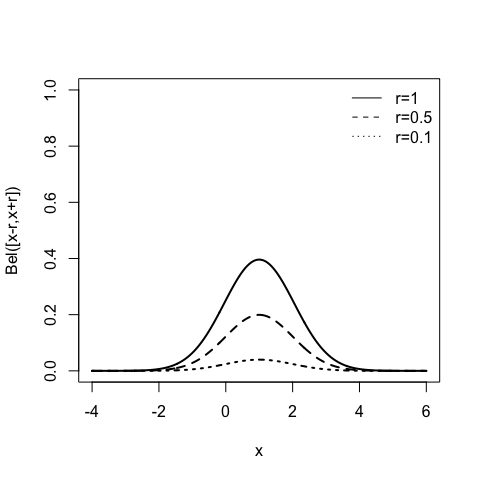and the “plausibility plot”: plot(x,Pl(x-1,x+1,GRFN),type="l",xlab="x",ylab="Pl([x-r,x+r])",lwd=2,ylim=c(0,1)) lines(x,Pl(x-0.5,x+0.5,GRFN),lwd=2,lty=2) lines(x,pl_contour(x,GRFN),lwd=2,lty=3) legend("topright",legend=c("r=1","r=0.5","r=0"),lty=c(1,2,3),bty="n")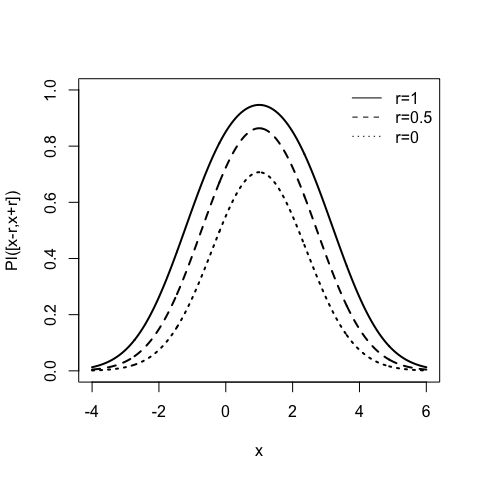We can also plot the lower cumulative distribution function (cdf) $$F_*(x)=Bel((-\infty,x])$$ and the upper cdf $$F^*(x)=Pl((-\infty,x])$$ as follows: plot(x,Bel(-Inf,x,GRFN),type="l",xlab="x",ylab="Lower/upper cdfs",lwd=2) lines(x,Pl(-Inf,x,GRFN),type="l",lwd=2)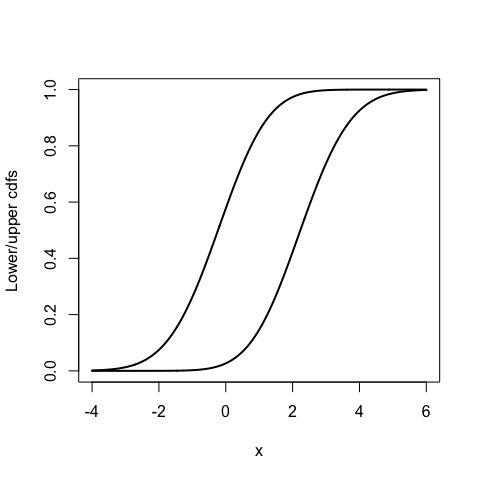Finally, evreg also has a function combination_GRFN that combines GRFNs using the generalized product-intersection rule with hard or soft normalization. The rule with soft normalization was introduced in (Denœux 2023a) and is recommended because it has very appealing properties (the contour function of the combined random fuzzy sets is still proportional to the product of the controur functions, a very useful property of Dempster’s rule preserved with soft normalization). However, the combination rule with hard normalization is simpler to compute and is implemented in the ENNreg model (Denœux 2023b). Here is an illustration of the use of function combination_GRFN with the two normalization schemes. Let us first define two GRFNs: GRFN1<-list(mu=0,sig=2,h=4) GRFN2<-list(mu=1,sig=1,h=1) and combine them with soft and hard normalization: GRFN12s<-combination_GRFN(GRFN1,GRFN2,soft=TRUE) GRFN12h<-combination_GRFN(GRFN1,GRFN2,soft=FALSE) print(GRFN12s$GRFN)
## $mu ##  0.68 ## ##$sig
##  1.077033
##
## $h ##  5 print(GRFN12h$GRFN)
## $mu ##  0.2 ## ##$sig
##  1.612452
##
## $h ##  5 We can see that the results are quite different. Here is a plot of the contour functions of the initial and combined GRFNs: x<-seq(-4,6,0.01) plot(x,pl_contour(x,GRFN1),type="l",xlab="x",ylab="plausibility",lwd=2, ylim=c(0,1),col="blue") lines(x,pl_contour(x,GRFN2),lwd=2,lty=1,col="red") lines(x,pl_contour(x,GRFN12s$GRFN),lwd=2,lty=2,col="green")
lines(x,pl_contour(x,GRFN12h\$GRFN),lwd=2,lty=2,col="cyan")
legend("topright",legend=c("GRFN1","GRFN2","soft comb.","hard comb."),
lty=c(1,1,2,2),bty="n",col=c("blue","red","green","cyan"))`